# Explanations supplement

This vignette provides additional documentation for some methods implemented in the emmeans package.

Index of all vignette topics

## Comparison arrows

The plot() method for emmGrid objects offers the option comparisons = TRUE. If used, the software attempts to construct “comparison arrows” whereby two estimated marginal means (EMMs) differ significantly if, and only if, their respective comparison arrows do not overlap. In this section, we explain how these arrows are obtained.

First, please understand these comparison arrows are decidedly not the same as confidence intervals. Confidence intervals for EMMs are based on the statistical properties of the individual EMMs, whereas comparison arrows are based on the statistical properties of differences of EMMs.

Let the EMMs be denoted $$m_1, m_2, ..., m_k$$. For simplicity, let us assume that these are ordered: $$m_1 \le m_2 \le \cdots \le m_k$$. Let $$d_{ij} = m_j - m_i$$ denote the difference between the $$i$$th and $$j$$th EMM. Then the $$(1 - \alpha)$$ confidence interval for the true difference $$\delta_{ij} = \mu_j - \mu_i$$ is $d_{ij} - e_{ij}\quad\mbox{to}\quad d_{ij} + e_{ij}$ where $$e_{ij}$$ is the “margin of error” for the difference; i.e., $$e_{ij} = t\cdot SE(d_{ij})$$ for some critical value $$t$$ (equal to $$t_{\alpha/2}$$ when no multiplicity adjustment is used). Note that $$d_{ij}$$ is statistically significant if, and only if, $$d_{ij} > e_{ij}$$.

Now, how to get the comparison arrows? These arrows are plotted with origins at the $$m_i$$; we have an arrow of length $$L_i$$ pointing to the left, and an arrow of length $$R_i$$ pointing to the right. To compare EMMs $$m_i$$ and $$m_j$$ (and remembering that we are supposing that $$m_i \le m_j$$), we propose to look to see if the arrows extending right from $$m_i$$ and left from $$m_j$$ overlap or not. So, ideally, if we want overlap to be identified with statistical non-significance, we want $R_i + L_j = e_{ij} \quad\mbox{for all } i < j$

If we can do that, then the two arrows will overlap if, and only if, $$d_{ij} < e_{ij}$$.

This is easy to accomplish if all the $$e_{ij}$$ are equal: just set all $$L_i = R_j = \frac12e_{12}$$. But with differing $$e_{ij}$$ values, it may or may not even be possible to obtain suitable arrow lengths.

The code in emmeans uses an ad hoc weighted regression method to solve the above equations. We give greater weights to cases where $$d_{ij}$$ is close to $$e_{ij}$$, because those are the cases where it is more critical that we get the lengths of the arrows right. Once the regression equations are solved, we test to make sure that $$R_i + L_j < d_{ij}$$ when the difference is significant, and $$\ge d_{ij}$$ when it is not. If one or more of those checks fails, a warning is issued.

That’s the essence of the algorithm. Note, however, that there are a few complications that need to be handled:

• For the lowest EMM $$m_1$$, $$L_1$$ is completely arbitrary because there are no right-pointing arrows with which to compare it; in fact, we don’t even need to display that arrow. The same is true of $$R_k$$ for the largest EMM $$m_k$$. Moreover, there could be additional unneeded arrows when other $$m_i$$ are equal to $$m_1$$ or $$m_k$$.
• Depending on the number $$k$$ of EMMs and the number of tied minima and maxima, the system of equations could be under-determined, over-determined, or just right.
• It is possible that the solution could result in some $$L_i$$ or $$R_j$$ being negative. That would result in an error.

In summary, the algorithm does not always work (in fact it is possible to construct cases where no solution is possible). But we try to do the best we can. The main reason for trying to do this is to enourage people to not ever use confidence intervals for the $$m_i$$ as a means of testing the comparisons $$d_{ij}$$. That is almost always incorrect.

What is better yet is to simply avoid using comparison arrows altogether and use pwpp() or pwpm() to display the P values directly.

### Examples and tests

Here is a constructed example with specified means and somewhat unequal SEs

m = c(6.1, 4.5, 5.4,    6.3, 5.5, 6.7)
se2 = c(.3, .4, .37,  .41, .23, .48)^2
lev = list(A = c("a1","a2","a3"), B = c("b1", "b2"))
foo = emmobj(m, diag(se2), levels = lev, linfct = diag(6))
plot(foo, CIs = FALSE, comparisons = TRUE)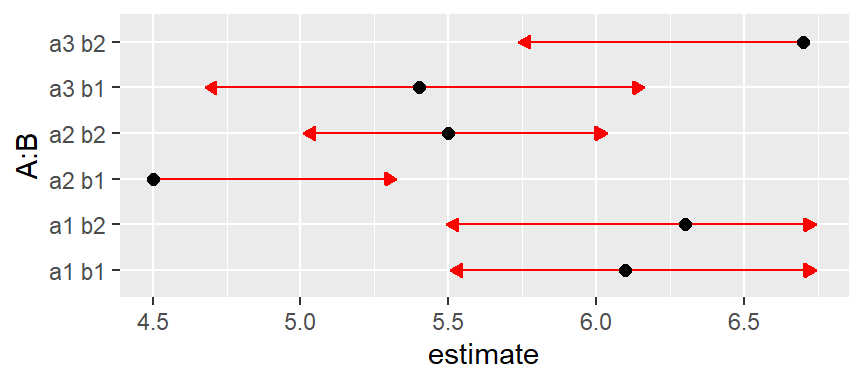This came out pretty well. But now let’s keep the means and SEs the same but make them correlated. Such correlations happen, for example, in designs with subject effects. The function below is used to set a specified intra-sclass correlation, treating A as a within-subjects (or split-plot) factor and B as a between-subjects (whole-plot) factor. We’ll start with a corelation of 0.3.

mkmat <- function(V, rho = 0, indexes = list(1:3, 4:6)) {
sd = sqrt(diag(V))
for (i in indexes)
V[i,i] = (1 - rho)*diag(sd[i]^2) + rho*outer(sd[i], sd[i])
V
}
# Intraclass correrlation = 0.3
foo3 = foo
foo3@V <- mkmat(foo3@V, 0.3)
plot(foo3, CIs = FALSE, comparisons = TRUE)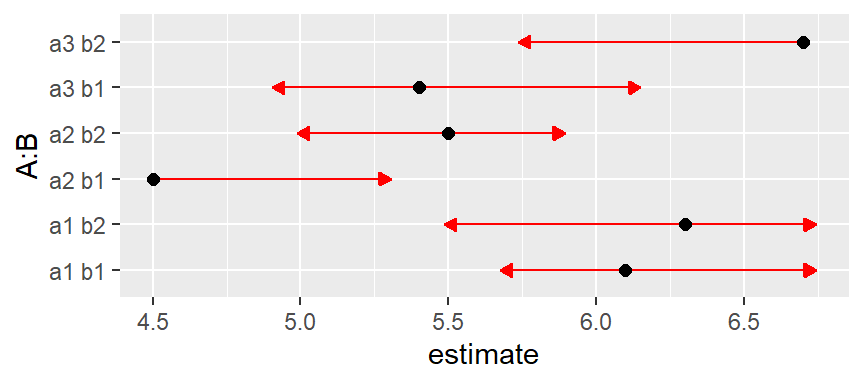Same with intraclass correlation of 0.6:

foo6 = foo
foo6@V <- mkmat(foo6@V, 0.6)
plot(foo6, CIs = FALSE, comparisons = TRUE)
## Warning: Comparison discrepancy in group 1, a1 b1 - a2 b2:
##     Target overlap = 0.443, overlap on graph = -0.2131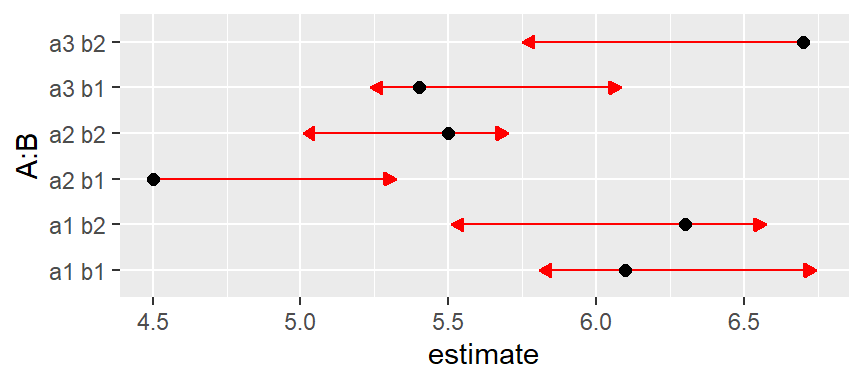Now we have a warning that some arrows don’t overlap, but should. We can make it even worse by upping the correlation to 0.8:

foo8 = foo
foo8@V <- mkmat(foo8@V, 0.8)
plot(foo8, CIs = FALSE, comparisons = TRUE)
## Error: Aborted -- Some comparison arrows have negative length!

Now the solution actually leads to negative arrow lengths.

What is happening here is we are continually reducing the SE of within-B comparisons while keeping the others the same. These all work out if we use B as a by variable:

plot(foo8, CIs = FALSE, comparisons = TRUE, by = "B")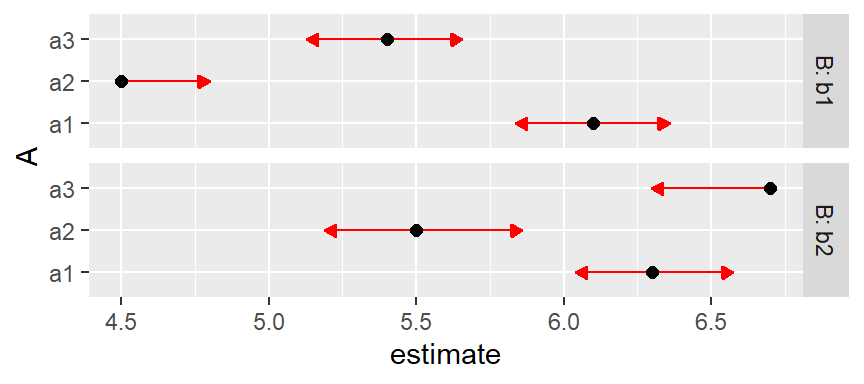Note that the lengths of the comparison arrows are relatively equal within the levels of B. Or, we can use pwpp() or pwpm() to show the P values for all comparisons among the six means:

pwpp(foo6, sort = FALSE)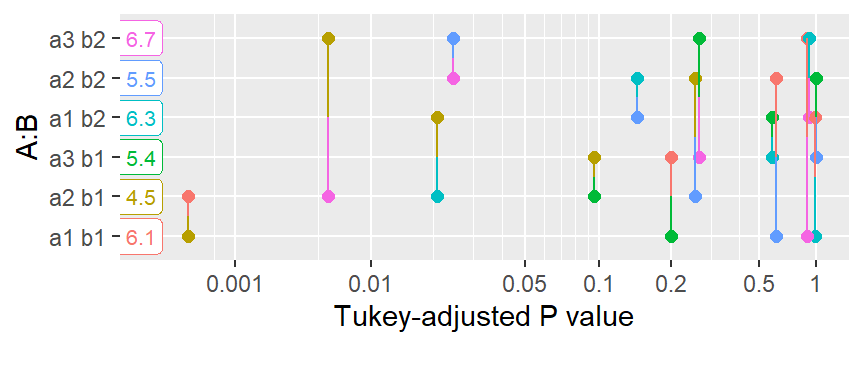pwpm(foo6)
##       a1 b1  a2 b1  a3 b1  a1 b2  a2 b2  a3 b2
## a1 b1 [6.1] <.0001 0.1993 0.9988 0.6070 0.8972
## a2 b1   1.6  [4.5] 0.0958 0.0208 0.2532 0.0057
## a3 b1   0.7   -0.9  [5.4] 0.5788 0.9999 0.2641
## a1 b2  -0.2   -1.8   -0.9  [6.3] 0.1439 0.9204
## a2 b2   0.6   -1.0   -0.1    0.8  [5.5] 0.0245
## a3 b2  -0.6   -2.2   -1.3   -0.4   -1.2  [6.7]
##
## Row and column labels: A:B
## Upper triangle: P values   adjust = "tukey"
## Diagonal: [Estimates] (estimate)
## Lower triangle: Comparisons (estimate)   earlier vs. later

Back to Contents

Index of all vignette topics